# NCERT Solutions for Class 6 Maths Chapter 6 Integers

## NCERT Solutions for Class 6 Maths Chapter 6 Integers

Today in our article you will be told that when your ncert solutions for class 6 maths Chapter 4 Basic Geometrical Ideas will be released and you will be provided complete information about it. In our article, you will also be given complete information about the model paper. Also, you will be provided complete information about the model paper download process in our article. So please read it carefully till the end and stay connected with our website till the end.

ncert solutions for class 6 maths Chapter 6 Integers

## Exercise 6.1

1. Write opposites of the following:

(a) Increase in weight

(b) 30 km north

(c) 80 m east

(d) Loss of Rs 700

(e) 100 m above sea level

Solutions:

(a) The opposite of an increase in weight is a decrease in weight

(b) The opposite of 30 km north is 30 km south

(c) The opposite of 80 m east is 80 m west

(d) The opposite of a loss of Rs 700 is a gain of Rs 700

(e) The opposite of 100 m above sea level is 100 m below sea level

2. Represent the following numbers as integers with appropriate signs.

(a) An airplane is flying at a height of two thousand meters above the ground.

(b) A submarine is moving at a depth, eight hundred meters below sea level.

(c) A deposit of rupees two hundred.

(d) Withdrawal of rupees seven hundred.

Solutions:

(a) + 2000 m

(b) – 800 m

(c) + Rs 200

(d) – Rs 700

3. Represent the following numbers on a number line:

(a) + 5

(b) – 10

(c) + 8

(d) – 1

(e) – 6

Solutions:

(a) + 5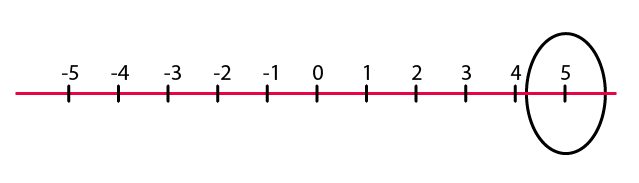(b) – 10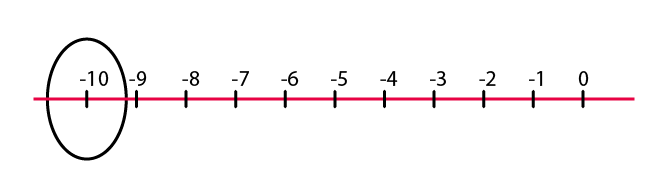(c) + 8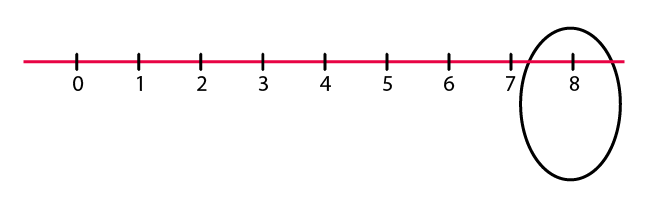(d) – 1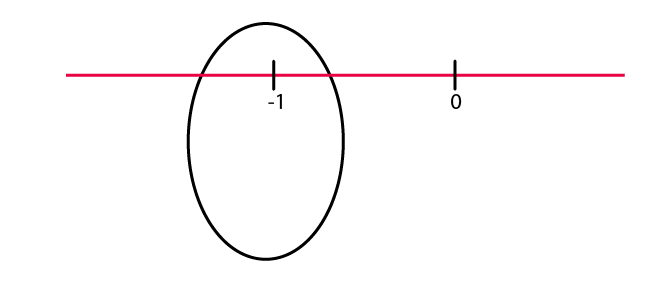(e) – 6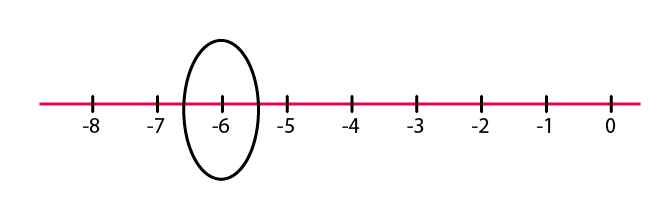4. Adjacent figure is a vertical number line, representing integers. Observe it and locate the following points:

(a) If point D is + 8, then which point is – 8?

(b) Is point G a negative integer or a positive integer?

(c) Write integers for points B and E.

(d) Which point marked on this number line has the least value?

(e) Arrange all the points in decreasing order of value.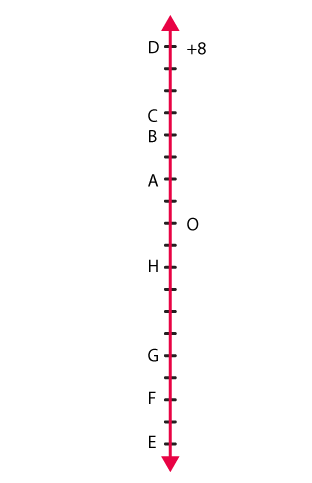Solutions:

(a) If point D is +8, then point F is -8

(b) Point G is a negative integer

(c) Point B is 4 and point E is – 10

(d) The least value on this number line is point E as it represents – 10

(e) The points in decreasing order of value are D, C, B, A, O, H, G, F, E

5. Following is the list of temperatures of five places in India on a particular day of the year.

Place Temperature

Siachin 10°C below 0°C ……………..

Shimla 2°C below 0°C ……………..

Delhi 20°C above 0°C ……………..

Srinagar 5°C below 0°C ……………..

(a) Write the temperatures of these places in the form of integers in the blank column.

(b) Following is the number line representing the temperature in degree Celsius.

Plot the name of the city against its temperature.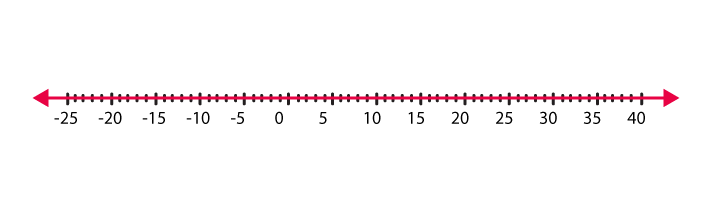(c) Which is the coolest place?

(d) Write the names of the places where temperatures are above 10°C.

Solutions:

(a)

Siachin – 100 C

Shimla – 20 C

Delhi + 200 C

Srinagar – 50 C

(b)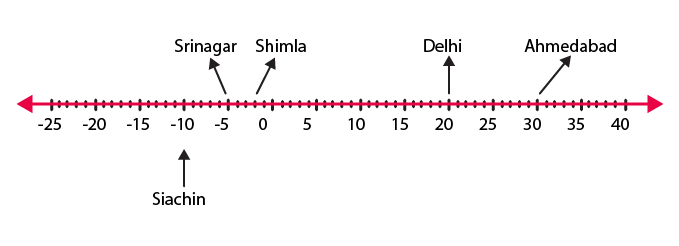(c) Siachin is the coolest place

(d) Ahmedabad and Delhi are the places where the temperatures are above 100C

6. In each of the following pairs, which number is to the right of the other on the number line?

(a) 2, 9

(b) – 3, – 8

(c) 0, – 1

(d) – 11, 10

(e) – 6, 6

(f) 1, – 100

Solutions:

(a) 9 lies to the right on the number line (9 > 2)

(b) – 3 lies to the right on the number line (- 3 > – 8)

(c) 0 lies to the right on the number line (0 > -1)

(d) 10 lies to the right on the number line (10 > -11)

(e) 6 lies to the right on the number line (6 > -6)

(f) 1 lies to the right on the number line (1 > -100)

7. Write all the integers between the given pairs (write them in increasing order.)

(a) 0 and – 7

(b) – 4 and 4

(c) – 8 and – 15

(d) – 30 and – 23

Solutions:

(a) -6, -5, -4, -3, -2, -1 are the integers between 0 and -7

(b) -3, -2, -1, 0, 1, 2, 3 are the integers between -4 and 4

(c) -14, -13, -12, -11, -10, -9 are the integers between -8 and -15

(d) -29, -28, -27, -26, -25, -24 are the integers between -30 and -23

8. (a) Write four negative integers greater than – 20.

(b) Write four integers less than – 10.

Solutions:

(a) -19, -18, -17, -16 are the integers greater then -20

(b) -11, -12, -13, -14 are the integers less than -10

9. For the following statements, write True (T) or False (F). If the statement is false, correct the statement.

(a) – 8 is to the right of – 10 on a number line.

(b) – 100 is to the right of – 50 on a number line.

(c) Smallest negative integer is – 1.

(d) – 26 is greater than – 25.

Solutions:

(a) True as (-8 > -10)

(b) False. (-50 is greater than -100). Hence, -100 lies to the left of -50 on the number line

(c) False. -1 is the greater negative integer.

(d) False. -26 is smaller than -25

10. Draw a number line and answer the following:

(a) Which number will we reach if we move 4 numbers to the right of – 2.

(b) Which number will we reach if we move 5 numbers to the left of 1.

(c) If we are at – 8 on the number line, in which direction should we move to reach – 13?

(d) If we are at – 6 on the number line, in which direction should we move to reach – 1?

Solutions:

(a)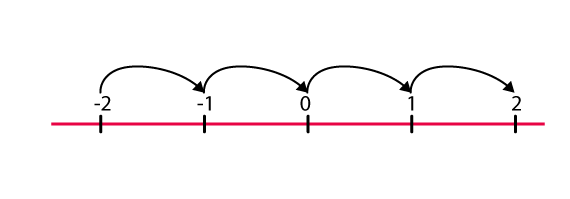If we move 4 numbers to the right of -2, we will reach at 2

(b)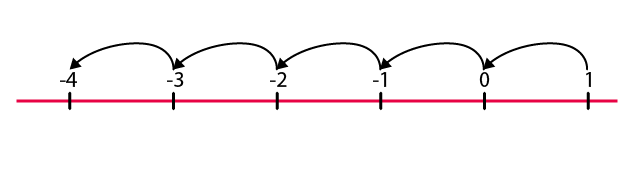If we move 5 numbers to the left of 1, we will reach at -4

(c)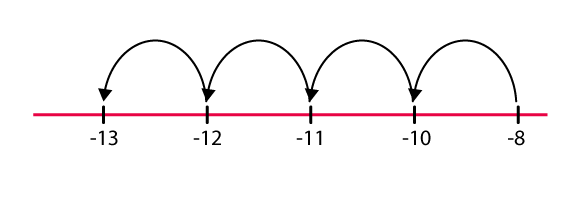-13 lies to the left of -8 on the number line. Hence, we should move towards the left direction

(d)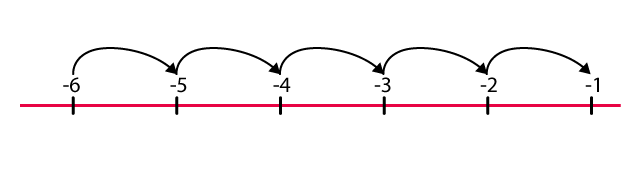-1 lies to the right of -6 on the number line. So, we should move in the right direction.

## Exercise 6.2

1. Using the number line write the integer which is:

(a) 3 more than 5

(b) 5 more than –5

(c) 6 less than 2

(d) 3 less than –2

Solutions:

(a)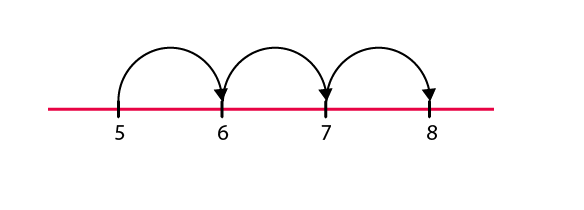Hence, 8

(b)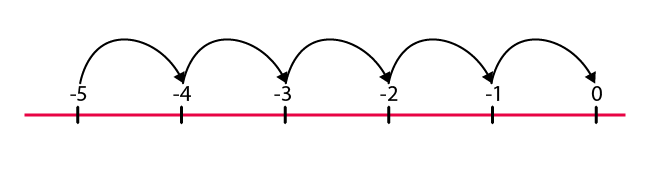Hence, 0

(c)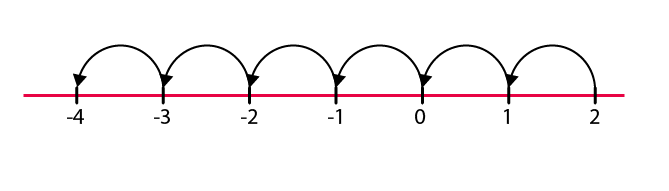Hence, -4

(d)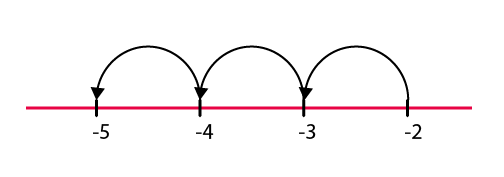Hence, -5

2. Use the number line and add the following integers:

(a) 9 + (–6)

(b) 5 + (–11)

(c) (–1) + (–7)

(d) (–5) + 10

(e) (–1) + (–2) + (–3)

(f) (–2) + 8 + (–4)

Solutions:

(a)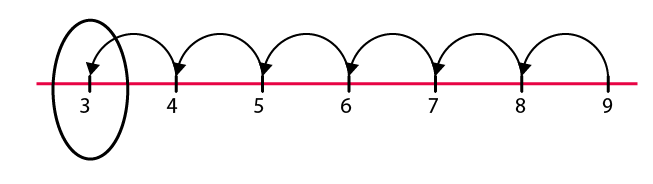Hence, 3

(b)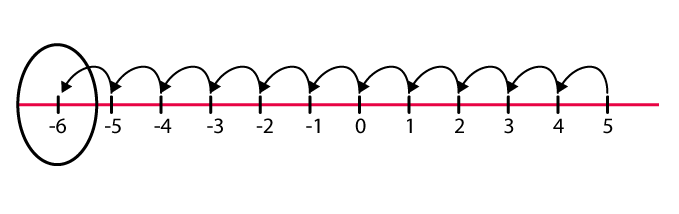Hence, -6

(c)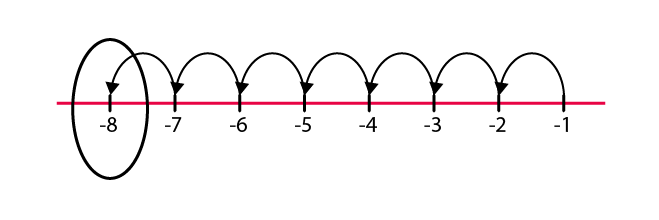Hence, -8

(d)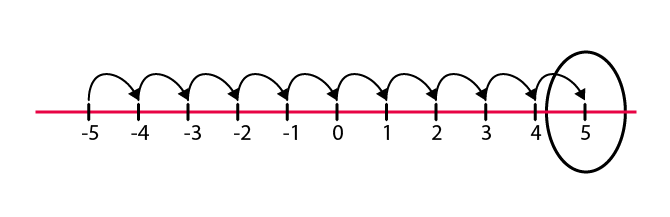Hence, 5

(e)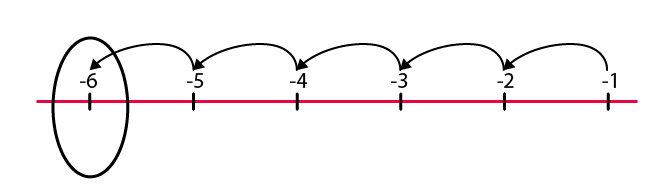Hence, -6

(f)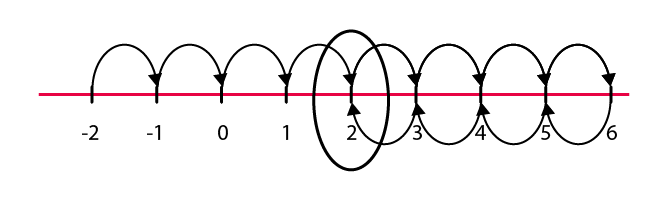Hence, 2

3. Add without using number line:

(a) 11 + (–7)

(b) (–13) + (+18)

(c) (–10) + (+19)

(d) (–250) + (+150)

(e) (–380) + (–270)

(f) (–217) + (–100)

Solutions:

(a) 11 + (-7) = 4

(b) (-13) + (+18) = 5

(c) (-10) + (+19) = 9

(d) (-250) + (+150) = -100

(e) (-380) + (-270) = -650

(f) (-217) + (-100) = -317

4. Find the sum of:

(a) 137 and – 354

(b) – 52 and 52

(c) – 312, 39 and 192

(d) – 50, – 200 and 300

Solutions:

(a) 137 and -354

(137) + (-354) = (137) + (-137) + (-217)

= 0 + (-217) [(137) + (-137) = 0]

= (-217)

= -217

(b) -52 and 52

(-52) + (+52) = 0 [(-a) + (+a) = 0]

(c) -312, 39 and 192

(-312) + (+39) + (+192) = (-231) + (-81) + (+39) + (+192)

= (-231) + (-81) + (+231)

= (-231) + (+231) + (-81)

= 0 + (-81) [(-a) + (+a) = 0]

= -81

(d) -50, -200 and 300

(-50) + (-200) + (+300) = (-50) + (-200) + (+200) + (+100)

= (-50) + 0 + (+100) [(-a) + (+a) = 0]

= (-50) + (+100)

= (-50) + (+50) + (+50)

= 0 + (+50) [(-a) + (+a) = 0]

= 50

5. Find the sum:

(a) (–7) + (–9) + 4 + 16

(b) (37) + (–2) + (–65) + (–8)

Solutions:

(a) (-7) + (-9) + 4 + 16

= (-7) + (-9) + 4 + (+7) + (+9)

= (-7) + (+7) + (-9) + (+9) + 4

= 0 + 0 + 4 [(-a) + (+a) = 0]

= 4

(b) (37) + (-2) + (-65) + (-8)

= (+37) + (-75)

= (+37) + (-37) + (-38)

= 0 + (-38) [(-a) + (+a) = 0]

= -38

## Exercise 6.3

1. Find

(a) 35 – (20)

(b) 72 – (90)

(c) (-15) – (-18)

(d) (-20) – (13)

(e) 23 – (-12)

(f) (-32) – (-40)

Solutions:

(a) 35 – (20)

35 – 20

15

(b) 72 – (90)

= 72 – 90

= -18

(c) (-15) – (-18)

= -15 + 18

= 3

(d) (-20) – (13)

= -20 – 13

= -33

(e) 23 – (-12)

= 23 + 12

= 35

(f) (-32) – (-40)

= -32 + 40

= 8

2. Fill in the blanks with >, < or = sign.

(a) (–3) + (–6) ______ (–3) – (–6)

(b) (–21) – (–10) _____ (–31) + (–11)

(c) 45 – (– 11) ______ 57 + (– 4)

(d) (–25) – (–42) _____ (– 42) – (–25)

Solutions:

(a) (-3) + (-6) = -9

(-3) – (-6) = -3 + 6 = 3

-9 < 3

Therefore (-3) + (-6) < (-3) – (-6)

(b) -21 – (-10) = -21 + 10 = -11

-31 + (-11) = -42

-11 > -42

Therefore (-21) – (-10) > (-31) + (-11)

(c) 45 – (-11) = 45 + 11 = 56

57 + (-4) = 57 – 4 = 53

56 > 53

Therefore 45 – (-11) > 57 + (-4)

(d) (-25) – (-42) = -25 + 42 = 17

-42 – (-25) = -42 + 25 = -17

17 > -17

Therefore (-25) – (-42) > (-42) – (-25)

3. Fill in the blanks.

(a) (–8) + _____ = 0

(b) 13 + _____ = 0

(c) 12 + (–12) = ____

(d) (–4) + ____ = –12

(e) ____ – 15 = – 10

Solutions:

(a) (-8) + 8 = 0

(b) 13 + (-13) = 0

(c) 12 + (-12) = 0

(d) (-4) + (-8) = -12

(e) 5 – 15 = -10

4. Find

(a) (–7) – 8 – (–25)

(b) (–13) + 32 – 8 – 1

(c) (–7) + (–8) + (–90)

(d) 50 – (–40) – (–2)

Solutions:

(a) (-7) – 8 – (-25) = -7 -8 + 25

= -15 + 25

= 10

(b) (-13) + 32 – 8 – 1 = -13 + 32 – 8 – 1

= 32 – 22

= 10

(c) (-7) + (-8) + (-90) = -7 – 8 – 90

= – 105

(d) 50 – (-40) – (-2) = 50 + 40 + 2

= 92

I hope you like the information and notes given by us. This will help you a lot in your upcoming exams. Here we are giving ncert solutions to all of you students, through which all of you can score very well in your exams. In this post, we are giving you ncert solutions for class 6 maths. hope you benefit from it. You can download the pdf file of this ncert solutions.

 Whatsapp Group Join
 Telegram channel Join